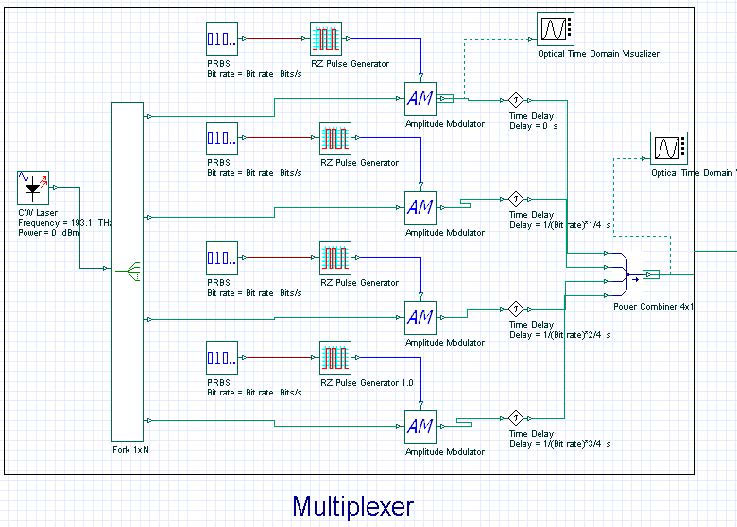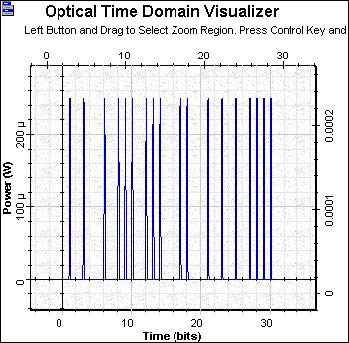# Time Division Multiplexing (TDM)

Compatibility:

In optical time-division multiplexing (OTDM) systems, several optical signal modulated at the bit rate B using the same carrier frequency are multiplexed optically to form a composite optical signal at a bit rate NB, where N is the number of multiplexed optical channels. OTDM Multiplexer.osd (see Figure 5) shows an OTDM transmitter.Figure 5: OTDM Multiplexer

Each modulator generates short pulses with 10 GB/s bit rate, the pulse 3 dB pulse width is 0.05 bit period (see Figure 6).

The demultiplexer  stage uses a modulator working at 10 GBs clock. Figure 8 shows the output signal as the first channel.Figure 8: OTDM 10 GBs-  Receiver